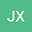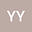loading page

On a class of inverse palindromic eigenvalue problem
••• Jiao Xu,
• Yongxin Yuan
Jiao Xu
Hubei Normal University
Author ProfileYongxin Yuan
Hubei Normal University
Author Profile## Abstract

In this paper we first give the general solution of the following inverse palindromic eigenvalue problem (IPEP): Given matrices $\Lambda= \mbox{diag}\{\lambda_{1}, \cdots, \lambda_{p}\} \in {\mathbb{C}}^{p\times p}$, $\lambda_{i}\neq \lambda_{j}$ for $i \neq j$, $i, j= 1, \cdots, p$, $X= [x_{1}, \cdots, x_{p}] \in {\mathbb{C}}^{n \times p}$ with $\mbox{rank}(X)= p$, and both $\Lambda$ and $X$ are closed under complex conjugation in the sense that $\lambda_{2i}= \bar{\lambda}_{2i-1} \in {\mathbb{C}}$, $x_{2i}= \bar{x}_{2i-1} \in {\mathbb{C}}^{n}$ for $i= 1, \cdots, m$, and $\lambda_{j} \in {\mathbb{R}}$, $x_{j} \in {\mathbb{R}}^{n}$ for $j= 2m+1, \cdots, p$, find a matrix $A \in {\mathbb{R}}^{n \times n}$ such that $AX= A^\top X\Lambda.$ We then consider a best approximation problem (BAP): Given $\tilde{A} \in {\mathbb{R}}^{n \times n}$, find $\hat{A} \in {\mathcal{S}}_{A}$ such that $\|\hat{A}-\tilde{A}\|= \min_{{A} \in {\mathcal{S}_{A}}} \|A-\tilde{A}\|,$ where $\|\cdot\|$ is the Frobenius norm and ${\mathcal{S}}_{A}$ is the solution set of IPEP. We show that the best approximation solution $\hat{A}$ is unique and derive an explicit formula for it.